# Voltage and Current Divider Rule (Formula & Example)

Contents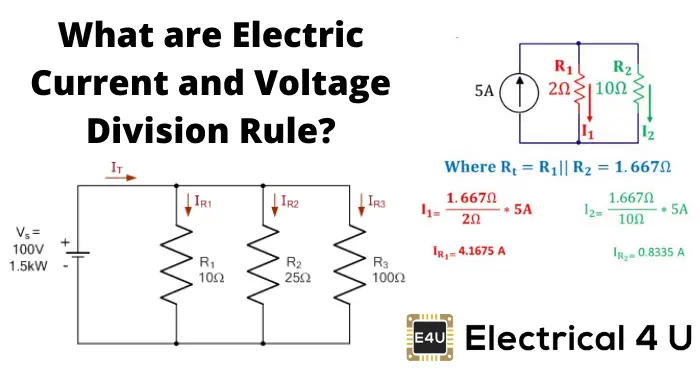## Current Division Rule

When current flows through more than one parallel path, each of the paths shares a definite portion of the total current depending upon the impedance of that path.

The definite portion of the total current shared by any of the parallel paths can easily be calculated if the impedance of that path and the equivalent impedance of the parallel system are known to us.

The rule or formula derived from these known impedances to know the portion of total current through any parallel path is known as the current divider rule. This rule is very important and widely used in the field of electric engineering in different applications.

Actually, this rule finds application when we have to find the current passing through each impedance when these are connected in parallel.

Let us say, two impedances Z1 and Z2 are connected in parallel as shown below.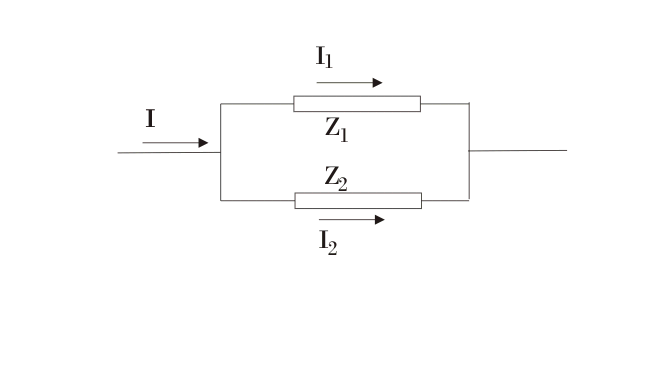A current I passes and is being divided into I1 and I2 at the junction of these two impedances as shown. I1 and I2 pass through Z1 and Z2 respectively. Our aim is to determine I1 and I2 in terms of I, Z1, and Z2.
As Z1 and Z2 are connected in parallel, the voltage drop across each will be the same. Hence, we can write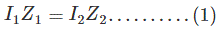Also applying Kirchoff’s current law at the junction, we get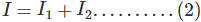We have two equations and can determine I1 and I2.
From (1), we have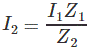Putting this in (2), we get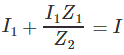or,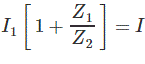or,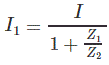or,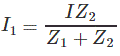We havePutting the value of I1, we get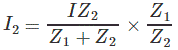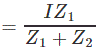Thus, we have determined I1 and I2 in terms of I, Z1, and Z2.

This rule is applied as follows.

Suppose we have to determine I1. We proceed as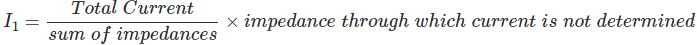Applying the above rule, we will get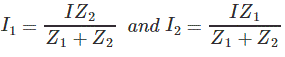Let us apply this rule to some problems.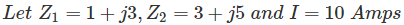Applying the current division rule, we will haveWhere I1 = current passing through Z1.

Putting given numerical values, we get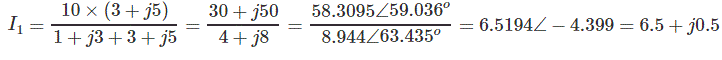Similarly,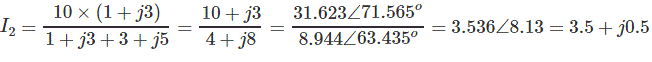The other way to find I2 is as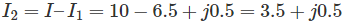This is how we can apply the current division rule.

## Voltage Division Rule

The voltage division rule is applied when we have to find the voltage across some impedance. Let us assume that the impedances Z1, Z2, Z3,…..Zn are connected in series, and the voltage source (V) is connected across them.

This is shown in the voltage divider circuit below:Our aim is to find the voltage across some impedance, say, Z3. We see that Z1, Z2, Z3 …. Zn are connected in series. Hence, effective impedance Zeff as seen by the voltage is given by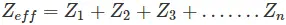The current passing the circuit is given by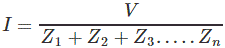This current is passing through all the impedances connected in series. Hence, the voltage across Z3 is given bySimilarly, the voltage across Z1 will be given by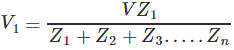In general, we can writeWhere, k = 1, 2, 3,….n and impedances Z1, Z2, Z3 ,…….Zn should be connected in series.

This is called the voltage division rule and frequently used to determine the voltage across some impedance. We can write this rule in words as given below.

The voltage across some impedanceWe will solve one problem of finding voltages across impedances using the voltage division rule.

### Voltage Division Rule Example Problem

The following impedances are connected in series: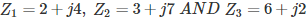Across this impedance connected in series, a voltage source of 100V is connected as shown below. Determine the voltage across each impedance.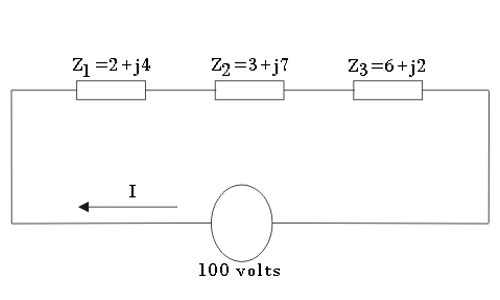Solution:
Applying the voltage division rule, we get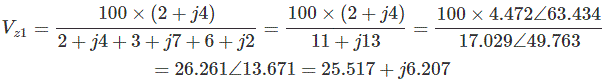Similarly,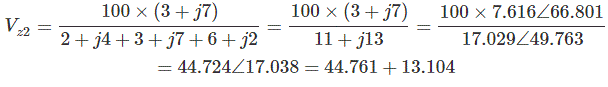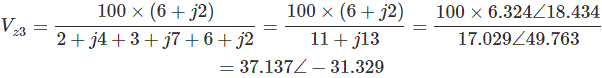We can also determine Vz3 as follows.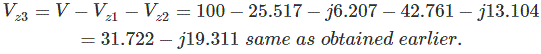Actually, we can determine the voltage across any impedance in this way if voltages across all other remaining impedances are known.

With the impedances equal to: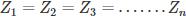The voltage across each impedance is given by: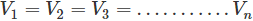Thus voltage will be the same across each impedance and it equals V/n, that is, source voltage divided by the number of impedances connected in series.

Want To Learn Faster? 🎓
Get electrical articles delivered to your inbox every week.
No credit card required—it’s 100% free.